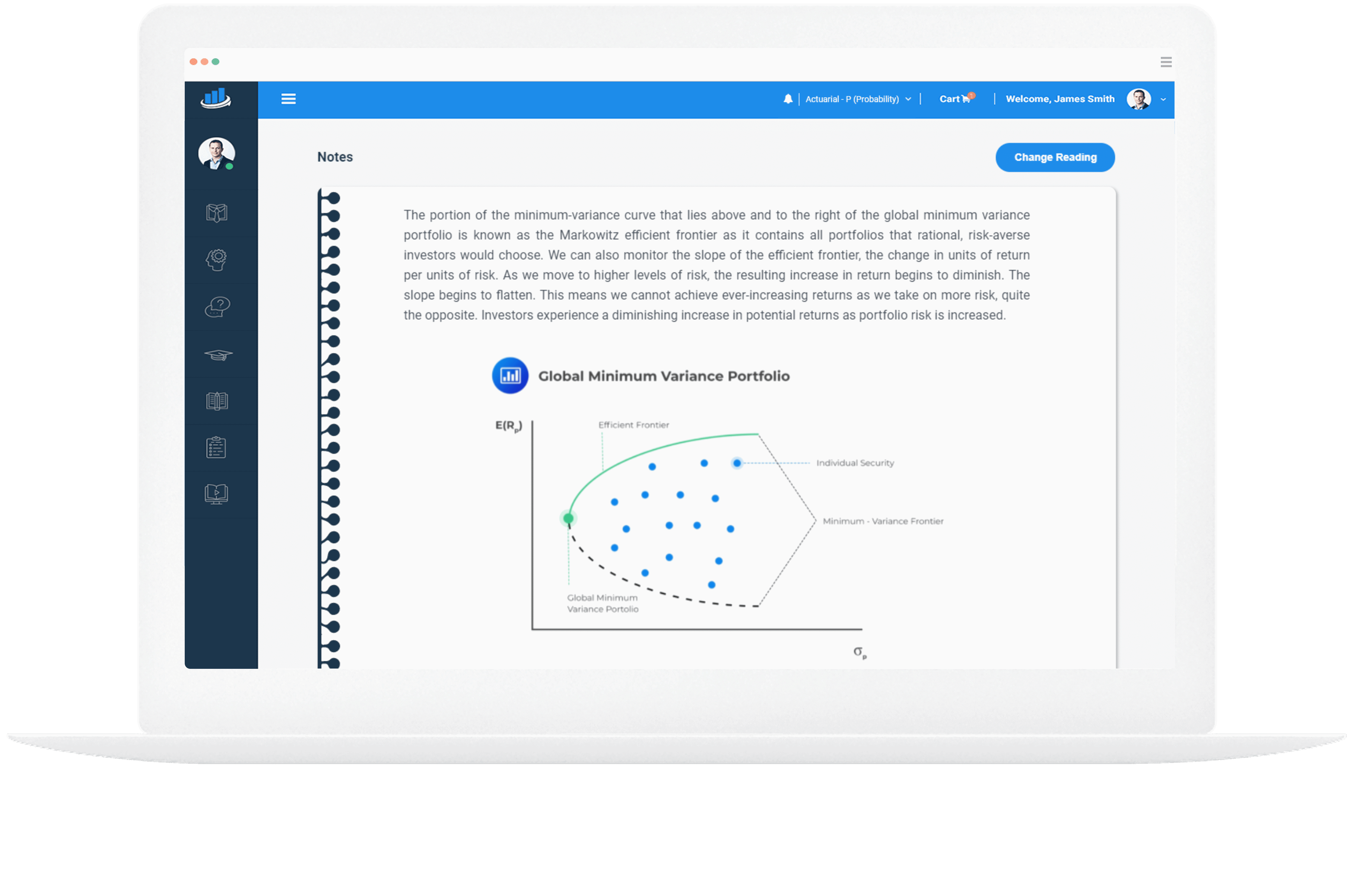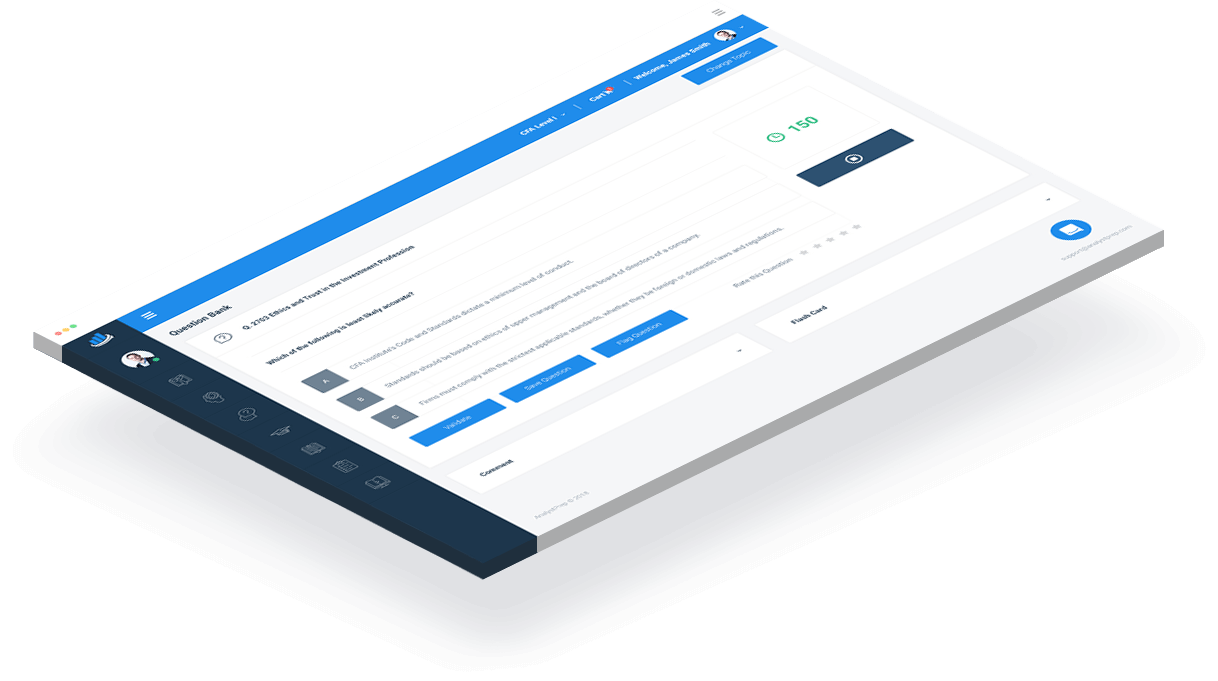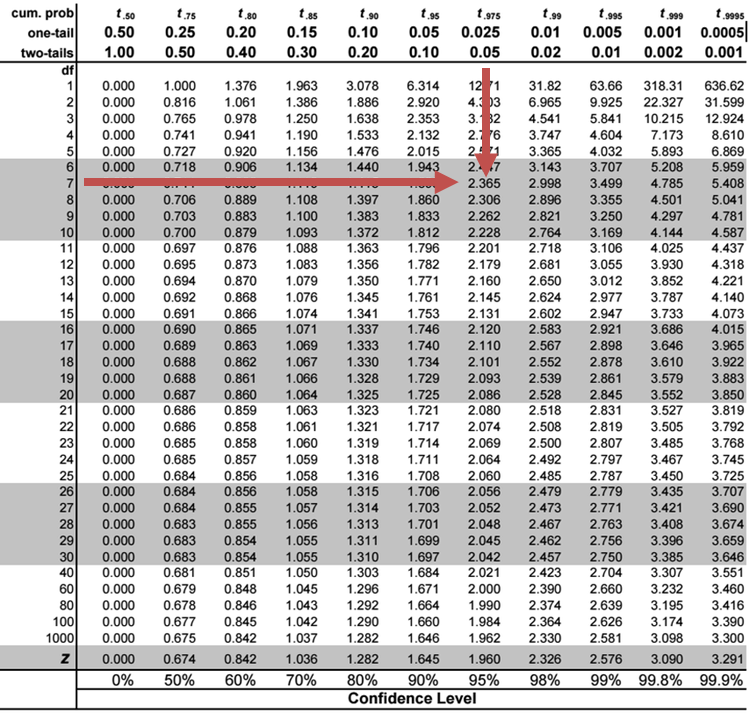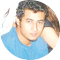Study Materials for 2023 CFA®, FRM®, Actuarial, GMAT® and EA® Exams

# Study Notes for Level II of the CFA® Exam

### Every Learning Outcome Statement (LOS) Summarized with Question Examples

The Level II study course consists of a vast amount of material in a 5,000-page CFA Institute official books that make up the curriculum. There are 10 topic areas that vary in emphasis and weight at each exam level. The amount of information to memorize and understand can be overwhelming. For this reason, we’ve produced comprehensive study notes in an easy-to-read format to help you learn efficiently and effectively.

According to CFA Institute, candidates require a minimum of 300 hours of study at each level and at least 6 months to prepare for Level II of the CFA exam. With statistics showing the pass rate hovering at 40% over the past 10 years, you need to perform better than the average candidate to secure a pass. In a 2015 CFA Institute survey, more than 60% of candidates admitted to using third-party preparation materials in addition to reading the assigned books.

4.5  Million
50  Thousand
# 1  Rated
###### Cfa Preparation Platform By Review Websites## Why Candidates Love AnalystPrep's Study Notes

Our study notes are designed and written by CFA charterholders to help you understand core concepts covered in the CFA exams in the most efficient way. We’ve produced summaries of each chapter from the latest CFA Program curriculum with clear, detailed explanations to help you understand difficult concepts and speed up your learning.

Spread out across the topics are handy tips and tricks to help you understand concepts faster. Our end-of-LOS questions help you to test your understanding of key concepts and build confidence. What’s more, our instructors are always ready to chip in with additional explanations whenever you need them.

## This isn't a Sprint

Many candidates have likened the CFA® exam experience to a marathon. We understand how exhausting it can get and want to help you get to the finishing line.

#### Stay on Course with our Study Plans

Plan a study schedule to fit your needs and busy lifestyle and stick to it. We’ll help you stay organized with our proposed one-month or two-month study plan and identify the chapters you should concentrate on. Remember, the chapters are not equally weighted in the evaluation.

#### Get Support Whenever you Need It

Our premium members enjoy the privilege of AnalystPrep’s community support. The experts from our support team are here to answer any of your exam related questions within 24 hours so you can stay on track and on schedule with your study plan.

Plus, our in-app forum connects you to a community of other CFA Program candidates, CFA charterholders, and AnaylstPrep founders, wherever you are and whenever you wish. This way, you stay motivated and engaged as you make the journey to your Level II CFA exam.### Example LOS from our Study Notes

#### Reading 2 – Multiple Regression

##### LOS 2 (c) Formulate a null and an alternative hypothesis about the population value of a regression coefficient, calculate the value of the test statistic, and determine whether to reject the null hypothesis at a given level of significance.

Hypothesis testing involves testing an assumption regarding a population parameter. A null hypothesis is a condition believed not to be true. We reject the null hypothesis in the presence of enough evidence against it and accept the alternative hypothesis.

Hypothesis testing is performed on the estimated slope coefficients to establish if the independent variables make a significant contribution to explaining the variation in the dependent variable.

The t-statistic for testing the significance of the individual coefficients in a multiple regression model is calculated using the formula:

$$t=\frac{\widehat{b_{j}}-b_{H0}}{S_{\widehat{b_{j}}}}$$

Where:

• $$\widehat{b_{j}}$$ = Estimated regression coefficient.
• $$b_{H0}$$ = Hypothesized value.
• $$S_{\widehat{b_{j}}}$$ The standard error of the estimated coefficient.

Note that the test statistic has $$n-k-1$$ degrees of freedom, where $$k$$ is the number of independent variables, and $$1$$ is the intercept term.

A t-test tests the null hypothesis that the regression coefficient is equal to some hypothesized value against the alternative hypothesis that it is not.

$$H_0:b_j=v$$ v.s $$H_{a}:b_{j}≠v$$

Where:

• $$v$$ = Hypothesized value.

The following are the steps followed in hypothesis testing:

i. Formulate a hypothesis.

• For example, Null hypothesis, $$H_0: b_{j}=0$$ vs Alternative hypothesis, $$H_{a}: b_{j}≠0$$.

ii. Determine the suitable test statistic.

$$t_{statistic}= \frac{\widehat{b_{j}}-b_{H0}}{S_{\widehat{b_{j}}}}$$

iii. Specify the significance level.

• 5% significance level is commonly used.

iv. Make the statistical decision.

• Reject the null hypothesis when the t-statistic is greater than the absolute value of the critical value of t.

#### Example: The Test of Significance for a Regression Coefficient

Consider the following multiple regression results of the price of USDX versur inflation rate and real interest rate.

$$\small{\begin{array}{l|c}\hline\textbf{Regression Statistics}\\ \hline\text{Multiple R} & 0.8264\\ \hline\text{R Square} & 0.6830\\ \hline\text{Adjusted R Square} & 0.5924\\ \hline\text{Standard Error} & 5.3537\\ \hline\text{Observations} & 10\\ \hline\end{array}}$$

$$\small{\begin{array}{l|c|c|c|c}{}& \textbf{Coefficients} & \textbf{Standard Error} & \textbf{t-statistic} & \textbf{P-value}\\ \hline\text{Intercept} & 81 & 7.9659 & 10.1296 & 0.0000\\ \hline\text{Inflation rates} & -276 & 233.0748 & -1.1833 & 0.2753\\ \hline\text{Real interest Rates} & 902 & 279.6949 & 3.2266 & 0.0145\\ \end{array}}$$

Test the statistical significance of the independent variable real rate of interest at the 5% significance level.

##### Solution

We are testing the hypothesis:

$$H_{0}$$: Real Interest Rate=0 versus $$H_{a}$$: Real Interest Rate ≠ 0.

The t-statistic is determined as:

$$t=\frac{\widehat{b_{j}}-b_{H0}}{S_{\widehat{b_{j}}}}$$

\begin{align*}t&=\frac{902-0}{279.6949}\\&=3.22\end{align*}

The 5% two-tailed critical t-value with $$10-2-1 = 7$$ degrees of freedom is 2.3646.Since the t-statistic, $$3.22$$, is greater than the critical value, $$2.365$$, we reject the null hypothesis and conclude that the real interest rate regression coefficient is statistically significantly different from zero at the 5% significance level.

## Question

Consider the following multiple regression results of the return on capital (ROC) vs. performance measures (profit margin (%), sales and debt ratio).

$$\small{\begin{array}{|l|c|}\hline\textbf{Regression Statistics}\\ \hline\text{Multiple R} & 0.7906\\ \hline\text{R Square} & 0.6251\\ \hline\text{Adjusted R Square} & 0.5715\\ \hline\text{Standard Error} & 1.1963\\ \hline\text{Observations} & 25\\ \hline\end{array}}$$

$$\small{\begin{array}{l|c|c|c|c|c}{}& \textbf{Coefficients} & \textbf{Standard Error} & \textbf{t Stat} & \textbf{P-value} \\ \hline\text{Intercept} & 8.6531 & 0.9174 & 9.4323 & 0.0000 \\ \hline \text{Sales} & 0.0009 & 0.0005 & 1.7644 & 0.0922\\ \hline\text{Debt ratio} & 0.0229 & 0.0165 & 1.3880 & 0.1797 \\ \hline\text{Profit Margin%} & 0.2996 & 0.0564 & 5.3146 & 0.0000\\ \end{array}}$$

Adil Suleman, CFA, wants to test whether $$b_{o}$$ is different from zero. Assuming a 5% significance level, the hypothesis used and the result of the hypothesis test are most likely:

$$\begin{array}{|l|l|l|} \hline \text{A} & H_{0}:b_{0}= 0, vs. H_{a}: b_0≠0 & {\text{Fail to reject the null hypothesis and conclude that}\\ b_0 \text{ is equal to zero.}}\\ \hline \text{B} & H_{0}:b_{0}= 0, vs. H_{a}: b_0=0 & {\text{Reject the null hypothesis and conclude that}\\ b_0 \text{ is equal to zero.}} \\ \hline \text{C} & H_{0}:b_{0}= 0, vs. H_{a}: b_0≠0 & {\text{Reject the null hypothesis and conclude that}\\ b_0 \text{ is not equal to zero.}} \\ \hline \end{array}$$

### Solution

The hypothesis will be structured as:

$$H_0:b_0$$, v.s. $$H_a: b_0 ≠0$$

$$\text{t-statistic}= 9.4323$$

This is a two-tailed test: The critical t-value with 23 degrees of freedom at the 5% significance level is ±2.069.

Notice that the t-stat is greater than the upper critical value. This prompts the rejection of the null hypothesis and conclusion that  is significantly different from zero.

LOS 2 (c) Formulate a null and an alternative hypothesis about the population value of a regression coefficient, calculate the value of the test statistic, and determine whether to reject the null hypothesis at a given level of significance.

### CFA® Program

#### Level II Study Packages by AnalystPrep

AnalystPrep's study packages for the Level II of the CFA Exam start as low as $249. Add video lessons and study notes for only$150 extra.

###### / 12-month access
• Question Bank
• CBT Mock Exams
• Performance Tracking Tools
##### Unlimited Package (All 3 Levels)
###### 699
• Question Banks for all three Levels of the CFA Exam
• Mock Exams for all three Levels of the CFA Exam
• Performance Tracking Tools
• Video Lessons for all three Levels of the CFA Exam
• Study Notes for all three Levels of the CFA Exam

### Testimonials

“Thanks to your program I passed the first level of the CFA exam, as I got my results today. You guys are the best. I actually finished the exam with 45 minutes left in [the morning session] and 15 minutes left in [the afternoon session]… I couldn’t even finish with more than 10 minutes left in the AnalystPrep mock exams so your exams had the requisite difficulty level for the actual CFA exam.”###### James B.

“I loved the up-to-date study materials and Question bank. If you wish to increase your chances of CFA exam success on your first attempt, I strongly recommend AnalystPrep.”###### Jose Gary

“Before I came across this website, I thought I could not manage to take the CFA exam alongside my busy schedule at work. But with the up-to-date study material, there is little to worry about. The Premium package is cheaper and the questions are well answered and explained. The question bank has a wide range of examinable questions extracted from across the whole syllabus. Thank you so much for helping me pass my first CFA exam.”###### Brian Masibo

“Good Day!
I cleared FRM Part I (May-2018) with 1.1.1.1. Thanks a lot to AnalystPrep and your support.
Regards,”@AnalystPrep provided me with the necessary volume of questions to insure I went into test day having in-depth knowledge of every topic I would see on the exams.”###### Justin T.

“Great study materials and exam-standard questions. In addition, their customer service is excellent. I couldn’t have found a better CFA exam study partner.”###### Joshua Brown

“I bought their FRM Part 1 package and passed the exam. Their customer support answered all of my questions when I had problems with what was written in the curriculum. I’m planning to use them also for the FRM Part 2 exam and Level I of the CFA exam.”###### Zubair Jatoi

“I bought the FRM exam premium subscription about 2 weeks ago. Very good learning tool. I contacted support a few times for technical questions and Michael was very helpful.”[]